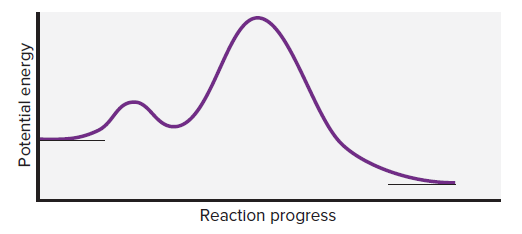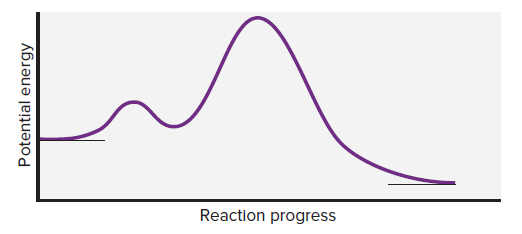# Problem: Consider the following reaction energy diagram:(a) How many elementary steps are in the reaction mechanism?

###### FREE Expert Solution

We’re being asked to determine the number of elementary steps in the reaction mechanism given.Let's label the given diagram:

96% (488 ratings)###### Problem Details

Consider the following reaction energy diagram:(a) How many elementary steps are in the reaction mechanism?

Frequently Asked Questions

What scientific concept do you need to know in order to solve this problem?

Our tutors have indicated that to solve this problem you will need to apply the Energy Diagram concept. If you need more Energy Diagram practice, you can also practice Energy Diagram practice problems.

What professor is this problem relevant for?

Based on our data, we think this problem is relevant for Professor Korolev's class at UF.

What textbook is this problem found in?

Our data indicates that this problem or a close variation was asked in Chemistry: The Molecular Nature of Matter and Change - Silberberg 8th Edition. You can also practice Chemistry: The Molecular Nature of Matter and Change - Silberberg 8th Edition practice problems.